# Cauchy principal value | Definite integral of 1/x

We know that the integral of 1/x is the natural logarithm ln(x). Very straightforward, yes, but it’s not as simple when it comes to evaluating the definite integral.

As you can see, at x=0, y tends to +infinity and -infinity. This is because 1/0 approaches infinity, and in this case we have two curves, one on the quadrant III, just before 0, and one on the quadrant I, just after 0, so we have: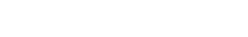0- and 0+ mean “just before” and “just after” 0. Think of them as -0.000…1 and +0.000…1.

If we are given this definite integral,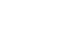we can solve it without any difficulty. If we look at the graph, we will see that from x=1 to x=3 there’s no divergency, i.e. no y values go to +/-infinity. This means that the integral is convergent, that is the result does not approach infinity. In other words, it is a finite number.

So, the integral of 1/x is ln(x) and we can plug in the values of x: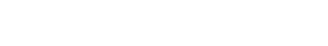But what if we have something like this: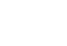Bad news! We can’t simply write ln|3|-ln|-2|. If we take a look at the graph of the function, we can see that, from -2 to 3, two values of y approach infinity (negative and positive). This means the integral is divergent.

So, how do we evaluate this integral? We need to use limits!

The problem here is that, since it goes from x=-2 to x=3, x=0 is included. We need to somehow “get rid of” the 0. The integral is defined from -2 to a number slightly before 0, which is 0-, and from a number slightly greater than 0, that is 0+. This means we have a sum of two integrals. Do you remember this property: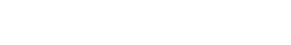?

a is -2 and c is 3. We said we have two integrals, one from -2 to 0- and one from 0+ to 3. b is the number that’s causing these trouble, 0, that we split into 0-, which is going to be our first b , and 0+, the second. Therefore we have: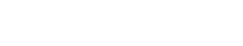But where are the limits I was talking about? Well, 0+ and 0- are used in limits, so the correct way to write this expression is: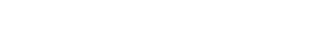This is called Cauchy principal value, and is a method used for evaluating definite integrals that would otherwise be undefined.

This is much better, since we have no 0’s. Now we can solve it the way we already know: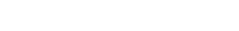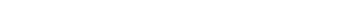We can now solve the limit by plugging in the values b tends to; remember that the result of the integral of 1/x is actually ln|x|, although ln(x) is acceptable. So the expression above becomes: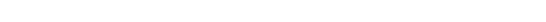Now, what is ln|0+| and ln|0-|? It’s like ln|0.0000000…1|, which tends to infinity, and ln|-0.0000000…1|, which tends to infinity as well, since the absolute value of -0.0000000…1 is 0.0000000…1 and ln(0.0000000…1) = infinity.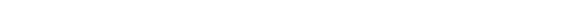ln(3)-ln(2) is out result. But wait… we also get this result when we plug the bounds into ln|x|, so what was the point of this? It can happen that the integral is divergent and the result is non finite, so you’ve got to use this method in order to figure it out.

This method is very useful and I hope that this lesson was interesting and helpful! If you have any questions leave a comment, and I’ll be happy to help! Also, if you liked this post, give it a like and subscribe for more! I would love any suggestions!

Join 31 other subscribers.